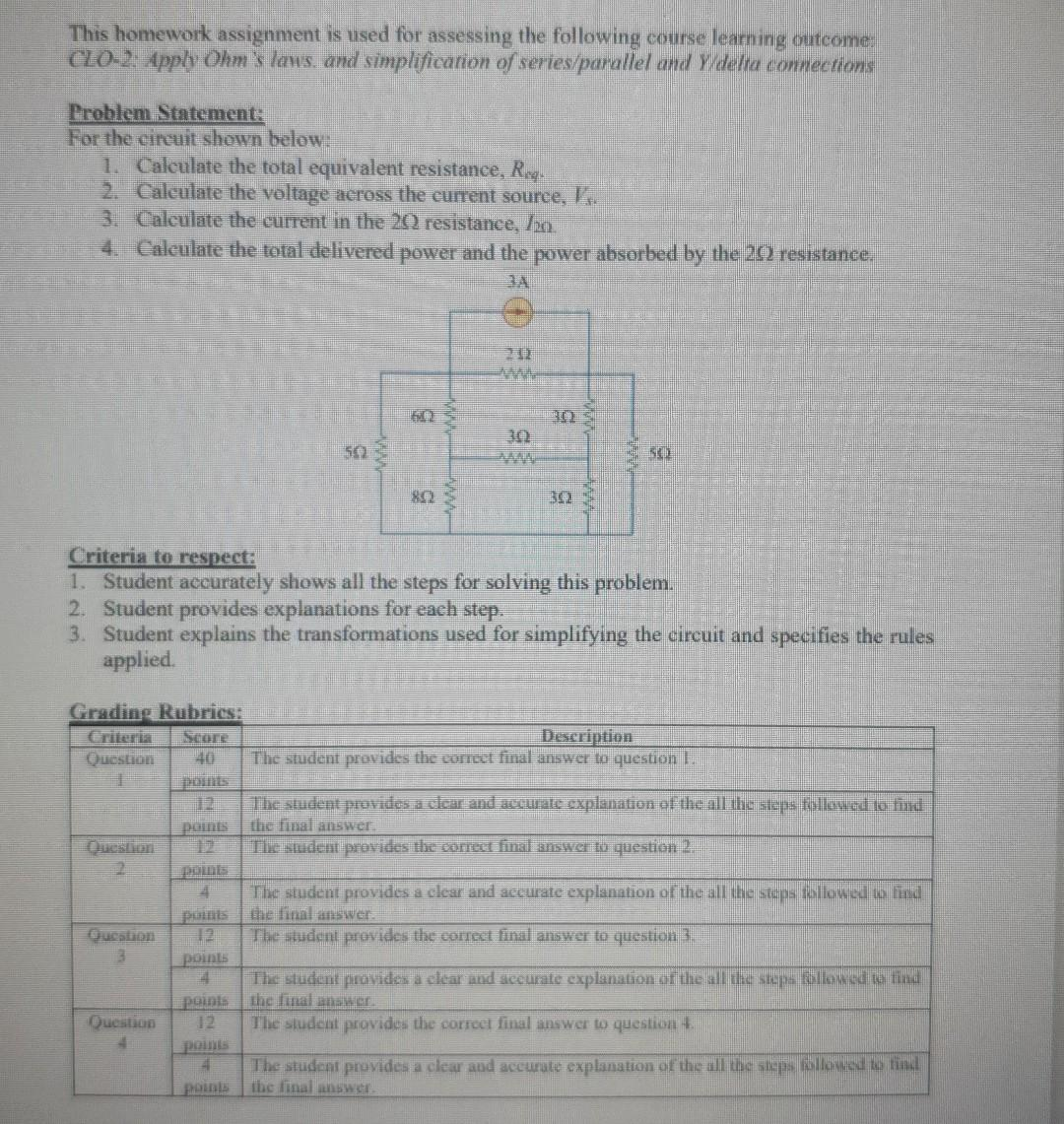# Question   hello , can you please solve it thanks in advance This homework assignment is used for assessing the following course learning outcome: CLO-2: Apply Ohm's laus, and simplificarion of seriesparallel and Y/delta connections Problem Statement: For the circuit shown below: 1. Caleulate the total equivalent resistance, $$R_{\text {rq . }}$$. 2. Calculate the voltage across the current source, $$V_{f}$$. 3. Calculate the current in the 20 resistance, $$/ 2 n$$ 4. Caleulate the total delivered power and the power absorbed by the $$2 \Omega 2$$ resistance. Criteria to respect: 1. Student accurately shows all the steps for solving this problem. 2. Student provides explanations for each step. 3. Student explains the transformations used for simplifying the circuit and specifies the rules applied.WONQD3 The Asker · Electrical Engineeringhello , can you please solve it

Transcribed Image Text: This homework assignment is used for assessing the following course learning outcome: CLO-2: Apply Ohm's laus, and simplificarion of seriesparallel and Y/delta connections Problem Statement: For the circuit shown below: 1. Caleulate the total equivalent resistance, $$R_{\text {rq . }}$$. 2. Calculate the voltage across the current source, $$V_{f}$$. 3. Calculate the current in the 20 resistance, $$/ 2 n$$ 4. Caleulate the total delivered power and the power absorbed by the $$2 \Omega 2$$ resistance. Criteria to respect: 1. Student accurately shows all the steps for solving this problem. 2. Student provides explanations for each step. 3. Student explains the transformations used for simplifying the circuit and specifies the rules applied.
Transcribed Image Text: This homework assignment is used for assessing the following course learning outcome: CLO-2: Apply Ohm's laus, and simplificarion of seriesparallel and Y/delta connections Problem Statement: For the circuit shown below: 1. Caleulate the total equivalent resistance, $$R_{\text {rq . }}$$. 2. Calculate the voltage across the current source, $$V_{f}$$. 3. Calculate the current in the 20 resistance, $$/ 2 n$$ 4. Caleulate the total delivered power and the power absorbed by the $$2 \Omega 2$$ resistance. Criteria to respect: 1. Student accurately shows all the steps for solving this problem. 2. Student provides explanations for each step. 3. Student explains the transformations used for simplifying the circuit and specifies the rules applied.